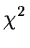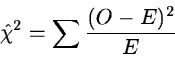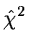Next: Multivariate Statistical Methods Up: Analysis of Results Previous: Regression Analysis

## Statistical Tests

Often, the computed estimators have to be compared with other values or model predictions. Different statistical tests are used to compute the probability of a given hypotheses being correct. A typical null hypotheses is that two quantities or samples are taken from the same population. For single quantities, a confidence interval is estimated for the desired significance level. The null hypotheses is then accepted at this level of significance if the value is within the interval. When the underlying distribution is normal, the Student's'' t and thedistributions are used to estimate the confidence intervals for the mean and standard deviation, respectively.

It is also possible to test if a set of observed values are taken from a given distribution. For this purpose the test variable(2.34)

is used where O and E are the observed and expected frequencies, respectively. The level of significance is derived fromwhich isdistributed. The bins must be so large that E is larger than 5 for all intervals.

When the underlying distribution is unknown, two independant samples can be compared using the Kolmogoroff and Smirnoff test. It uses the test variable(2.35)

where Fj is the cumulative frequency in the ith interval with nj values for the two samples j=1,2. The intervals must be of equal size and have the same limits for both samples. Special tables give the confidence interval for this test variable. Several other tests are available for comparing independent samples such as the U-test of Wilcoxon, Mann and Whitney which uses the rank in its test variable.Next: Multivariate Statistical Methods Up: Analysis of Results Previous: Regression Analysis
Petra Nass
1999-06-15